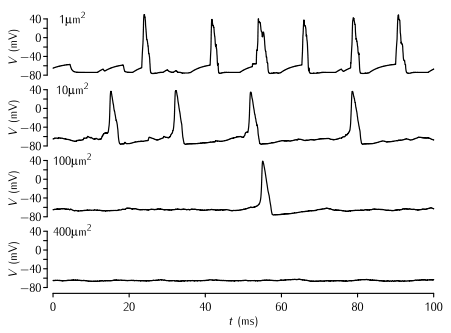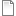# Figure 5.19Action potentials due to stochastic sodium and potassium channels

Simulations of patches of membrane of various sizes with stochastic Na+ and K+ channels. The density of Na+ and K+ channels is 60 channels per µm2 and 18 channels per µm2 respectively, and the single channel conductance of both types of channel γ = 2 pS. The Markov kinetic scheme versions of the Hodgkin-Huxley potassium and sodium channel models were used (Schemes 5.5 and 5.8). The leak conductance and capacitance is modelled as in the standard HH model. No current is applied; the action potentials are due to the random opening of a number of channels taking the membrane potential to  above threshold.

Simulation environment:
Notes

To produce a trace similar to the top trace in the figure:

1. In the Run Control window click on Init & Run.
2. A trace similar to the top one in the figure will appear in the top right graph. Below this is a graph of the sodium and potassium currents.

To reproduce each of the other panels from the figure:

1. In the soma window change the length L of the soma to the area of membrane you wish to simulate. Since the diameter of the neuron is 1/π the neuron's area in µm2 is equal to its length in µm.
2. In the PointProcessGroupManager window click on nahh. In the panel on the right set Nsingle to 18 multiplied by the area in µm2. For example if the area is 10µm2, Nsingle should be 180. This step is necessary because the NEURON PointProcess describes the number of channels inserted rather than their density. To maintain a constant density, the number of channels has to be scaled in line with the area.
3. In the PointProcessGroupManager window click on khh. In the panel on the right set Nsingle to 60 multiplied by the area in µm2. For example if the area is 10µm2, Nsingle should be 600.
4. In the Run Control window click on Init & Run. Traces should appear as above.

To investigate the kinetic schems of the sodium and potassium channels:

1. In the ChannelBuild window click on O: 8 state, 10 tranisitions. This shows the sodium kinetic scheme.
2. In the ChannelBuild window click on O: 5 state, 4 tranisitions. This shows the potassium kinetic scheme.fig-5-19.nrnzip